# How to Draw Bohr Diagrams and Lewis Diagrams

• Slides: 31How to Draw Bohr Diagrams and Lewis Diagrams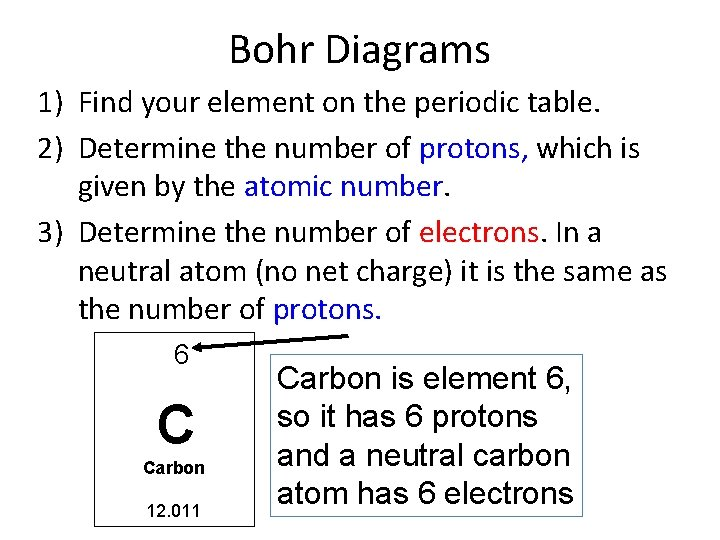Bohr Diagrams 1) Find your element on the periodic table. 2) Determine the number of protons, which is given by the atomic number. 3) Determine the number of electrons. In a neutral atom (no net charge) it is the same as the number of protons. 6 C Carbon 12. 011 Carbon is element 6, so it has 6 protons and a neutral carbon atom has 6 electrons6 p 6 n Bohr Diagrams 4) For carbon, write 6 p where your nucleus is going to be. 5) Next, figure out the number of neutrons. Protons and neutrons each have a mass of 1 atomic mass unit (a. m. u. ) Carbon’s atomic mass rounds to 12, so 12 – 6 protons = 6 neutrons Write 6 n 6)Draw a small circle around the numbers to indicate the nucleus.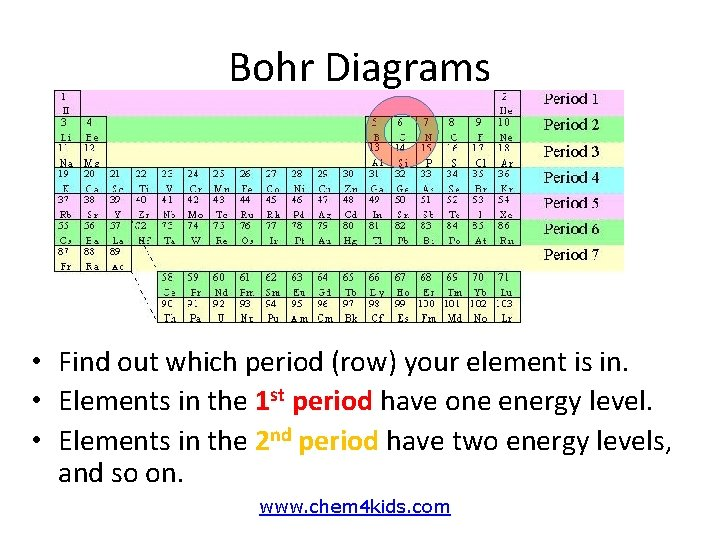Bohr Diagrams • Find out which period (row) your element is in. • Elements in the 1 st period have one energy level. • Elements in the 2 nd period have two energy levels, and so on. www. chem 4 kids. com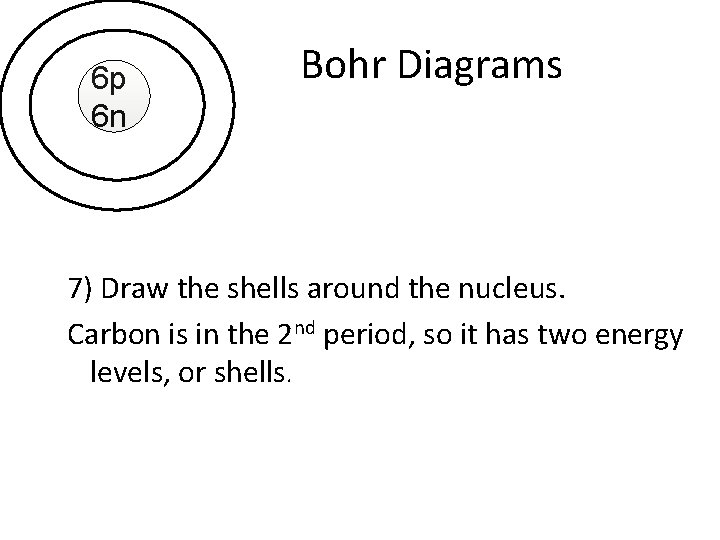6 p 6 n Bohr Diagrams 7) Draw the shells around the nucleus. Carbon is in the 2 nd period, so it has two energy levels, or shells.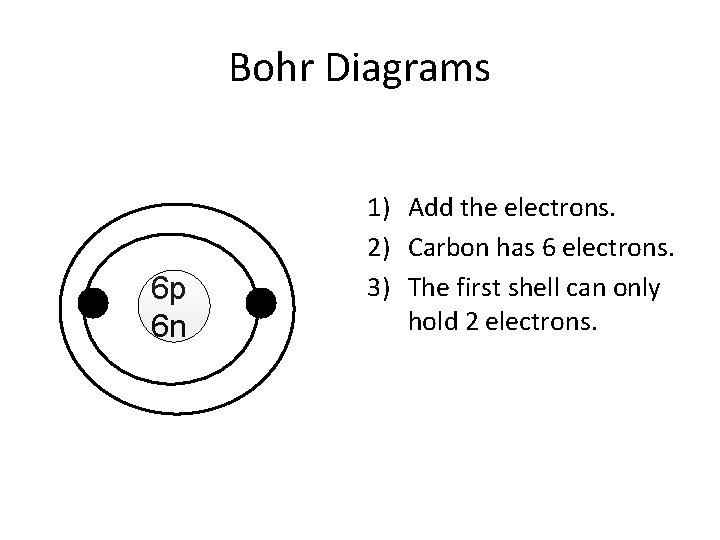Bohr Diagrams 6 p 6 n 1) Add the electrons. 2) Carbon has 6 electrons. 3) The first shell can only hold 2 electrons.Bohr Diagrams 6 p 6 n 1) Since you have 2 electrons already drawn, you need to add 4 more. 2) These go in the 2 nd shell. 3) Add one at a time starting on the right side and going counter clock-wise.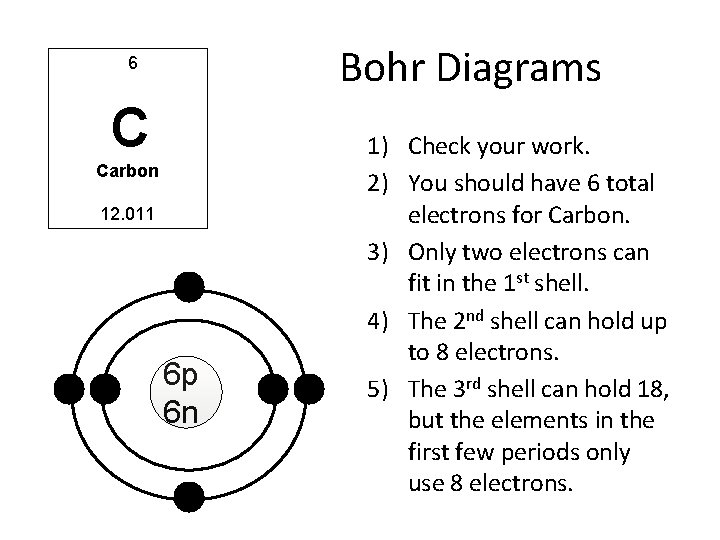Bohr Diagrams 6 C Carbon 12. 011 6 p 6 n 1) Check your work. 2) You should have 6 total electrons for Carbon. 3) Only two electrons can fit in the 1 st shell. 4) The 2 nd shell can hold up to 8 electrons. 5) The 3 rd shell can hold 18, but the elements in the first few periods only use 8 electrons.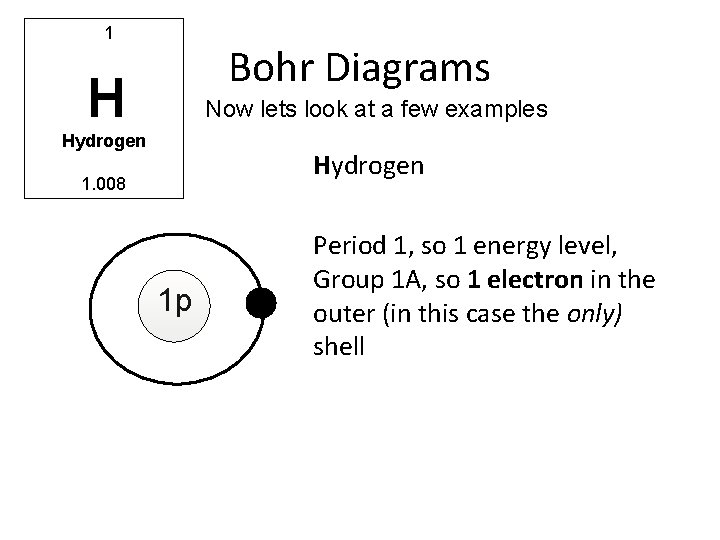1 Bohr Diagrams H Now lets look at a few examples Hydrogen 1. 008 1 p Period 1, so 1 energy level, Group 1 A, so 1 electron in the outer (in this case the only) shell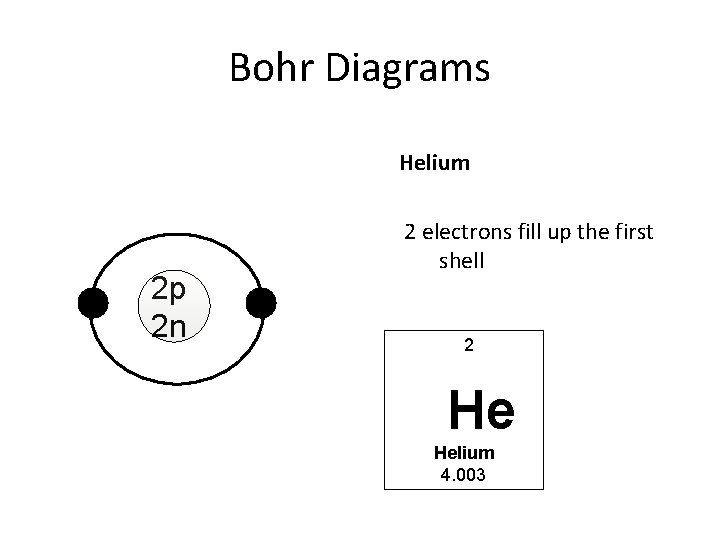Bohr Diagrams Helium 2 p 2 n 2 electrons fill up the first shell 2 He Helium 4. 003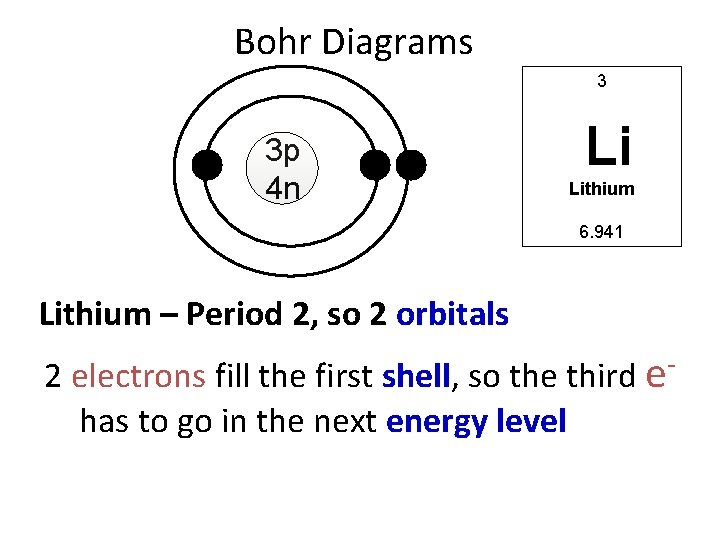Bohr Diagrams 3 3 p 4 n Li Lithium 6. 941 Lithium – Period 2, so 2 orbitals 2 electrons fill the first shell, so the third ehas to go in the next energy levelAs you add e- to the 2 nd shell, go counterclockwise through 3, 12, 9, and 6 o’clock positions. Li 3 p 4 n Each time you add an electron, draw a NEW Bohr diagram When with youtheget to and N (nitrogen) useinthe complete proton neutron information the nucleus the correct and element symbol. Follow the same. AND positions PAIR the electrons. example of Lithium, showing now. Click now to get started.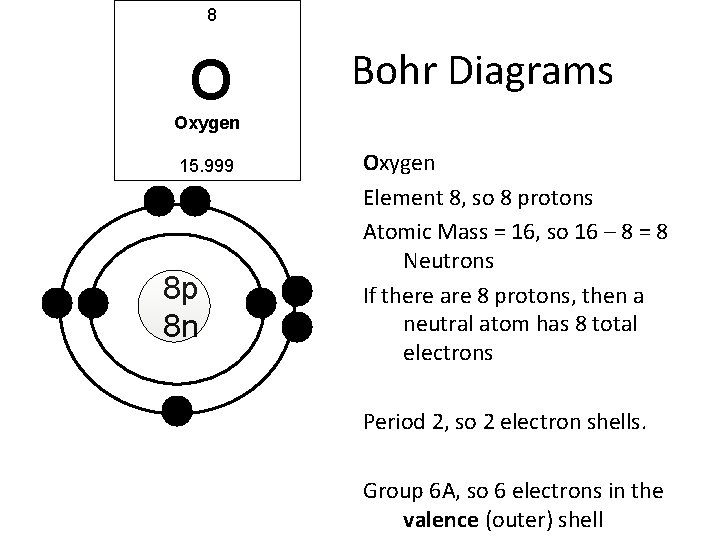8 O Bohr Diagrams Oxygen 15. 999 8 p 8 n Oxygen Element 8, so 8 protons Atomic Mass = 16, so 16 – 8 = 8 Neutrons If there are 8 protons, then a neutral atom has 8 total electrons Period 2, so 2 electron shells. Group 6 A, so 6 electrons in the valence (outer) shell10 Bohr Diagrams N Neon 20. 180 Neon 10 electrons 10 p n Period 2 = 2 shells Group 8 A = 8 valence electrons (making that shell full, so any more electrons will have to start a new shell…11 Bohr Diagrams Na Sodium 23. 990 11 electrons N p n Period 3 = 3 shells Group 1 A = 1 more electron than neon, so there is a new shell with 1 valence electron13 Al Aluminum 12. 011 Bohr Diagrams Aluminum 13 electrons total Period 3, so 3 electron shells Group 3 A, so 3 electrons in the valence shell (NOTICE that period 1 is full with 2 electrons and period 2 is full with 8 electrons)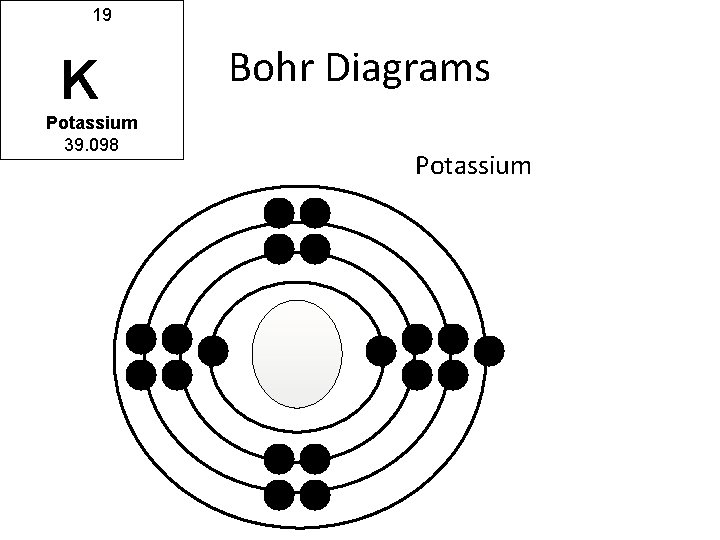19 K Potassium 39. 098 Bohr Diagrams Potassium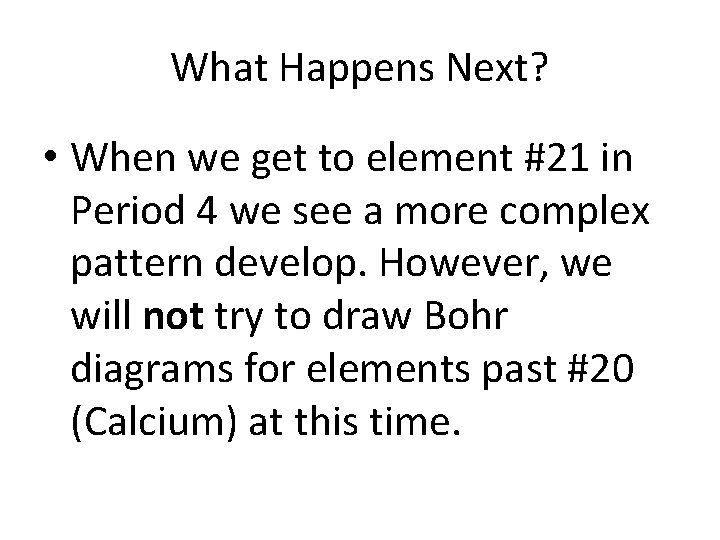What Happens Next? • When we get to element #21 in Period 4 we see a more complex pattern develop. However, we will not try to draw Bohr diagrams for elements past #20 (Calcium) at this time.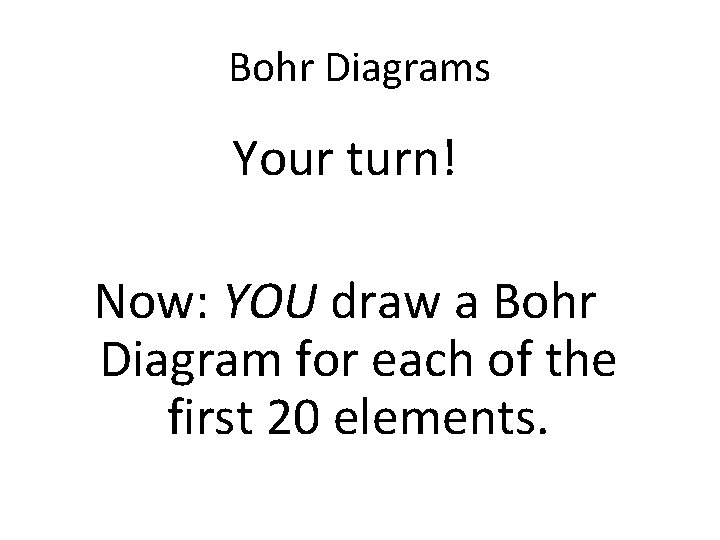Bohr Diagrams Your turn! Now: YOU draw a Bohr Diagram for each of the first 20 elements.Lewis Diagrams When we talk about atomic bonding in chemical reactions (atoms sticking together to make all the chemicals that exist) we just need to look at the outer or valence electrons. That’s where the atoms bond.For A group elements, the number of valence electrons is the same as the group number 1 A 1 8 A 2 A 2 Group number Number of Valence electrons 3 A 4 A 3 4 5 A 6 A 7 A 5 6 7 8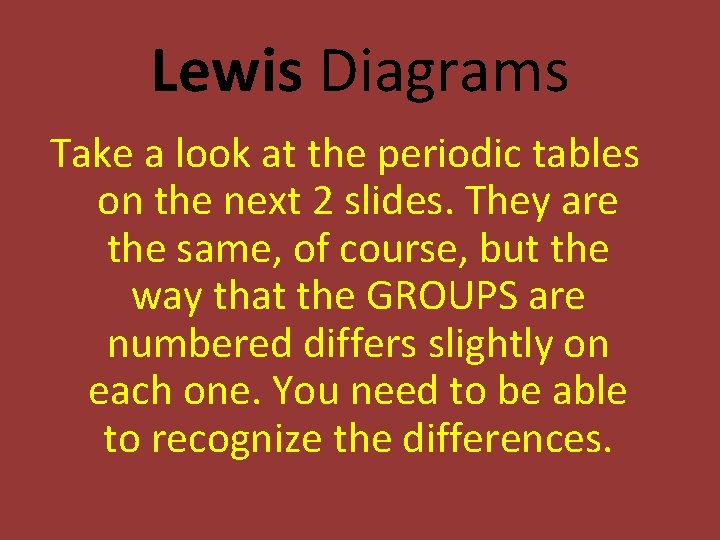Lewis Diagrams Take a look at the periodic tables on the next 2 slides. They are the same, of course, but the way that the GROUPS are numbered differs slightly on each one. You need to be able to recognize the differences.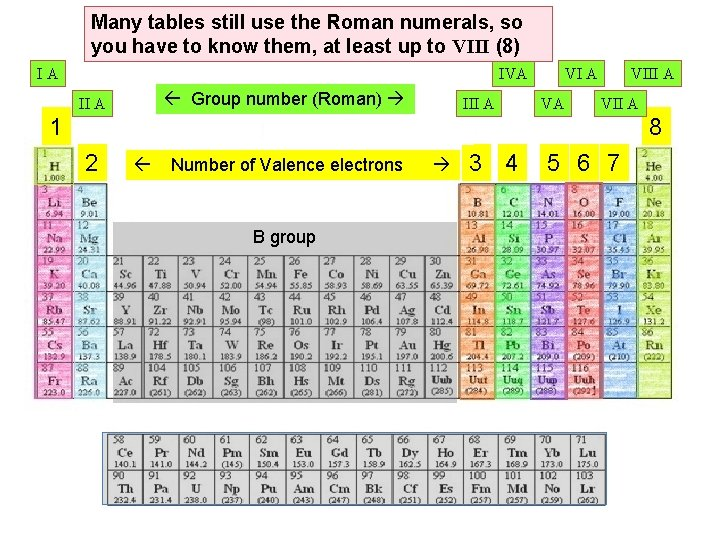Many tables still use the Roman numerals, so you have to know them, at least up to VIII (8) IA IVA Group number (Roman) II A III A VA VIII A VII A 1 8 2 Number of Valence electrons B group 3 4 5 6 7Some tables don’t separate A and B groups. Instead they just number all the groups 1 – 18. That’s no problem, because you just drop the “ 1” from the front of the number and you still have the A-group valence electron count! 1 1 18 2 2 13 Number of Valence electrons 3 Group number 4 5 6 7 8 9 10 11 12 14 3 4 15 16 17 5 6 7 8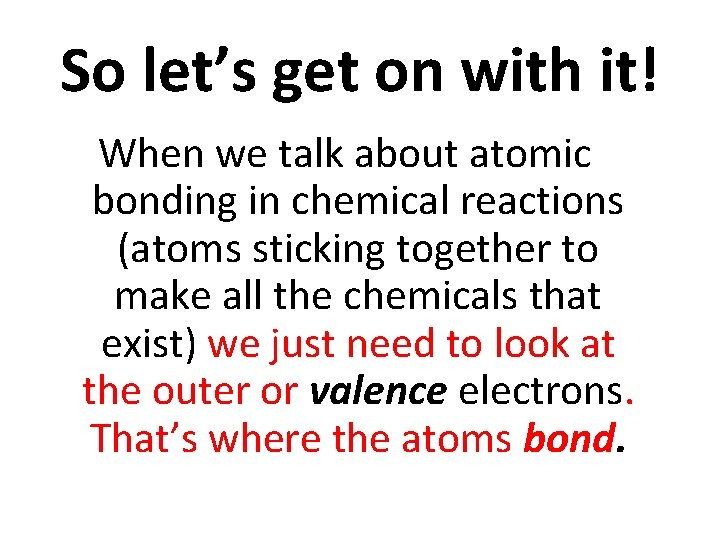So let’s get on with it! When we talk about atomic bonding in chemical reactions (atoms sticking together to make all the chemicals that exist) we just need to look at the outer or valence electrons. That’s where the atoms bond.Lewis Diagrams 6 C Carbon 12. 011 C 1) In this Bohr diagram of carbon, you see all of the electrons. 2) You only need to know about the valence shell to know how many bonds carbon can make 3) So, next we’ll take away the drawing of the first (inner) energy level. (It’s still there…we just don’t have to worry about it for bonding!)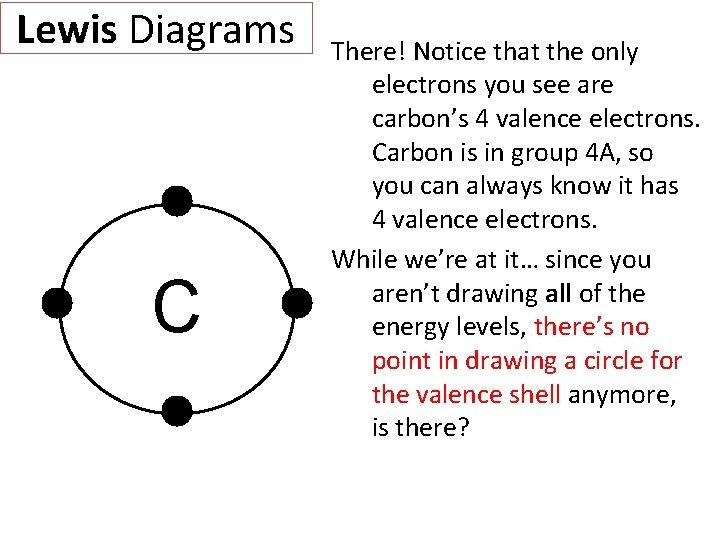Lewis Diagrams C There! Notice that the only electrons you see are carbon’s 4 valence electrons. Carbon is in group 4 A, so you can always know it has 4 valence electrons. While we’re at it… since you aren’t drawing all of the energy levels, there’s no point in drawing a circle for the valence shell anymore, is there?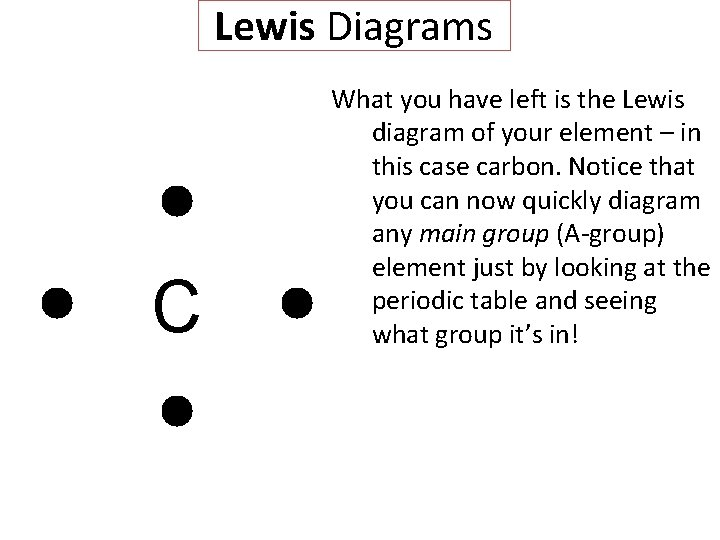Lewis Diagrams C What you have left is the Lewis diagram of your element – in this case carbon. Notice that you can now quickly diagram any main group (A-group) element just by looking at the periodic table and seeing what group it’s in!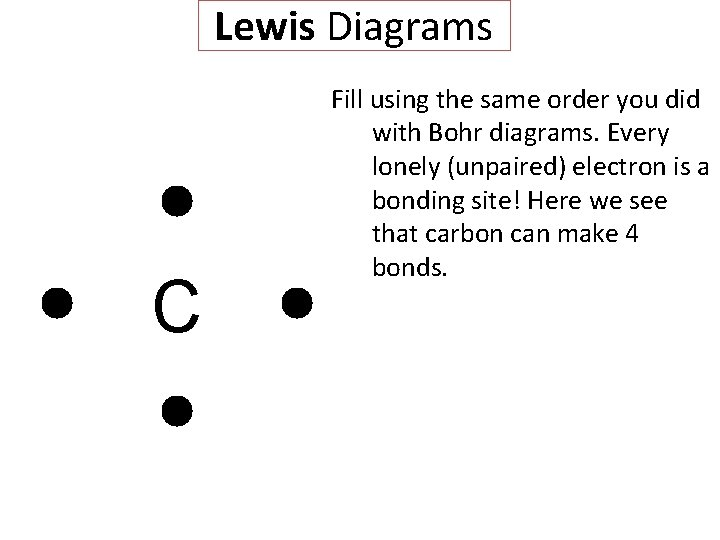Lewis Diagrams C Fill using the same order you did with Bohr diagrams. Every lonely (unpaired) electron is a bonding site! Here we see that carbon can make 4 bonds.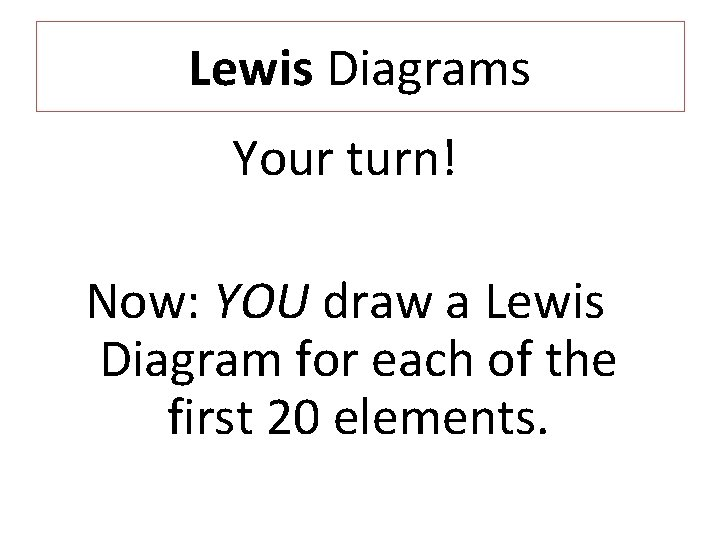Lewis Diagrams Your turn! Now: YOU draw a Lewis Diagram for each of the first 20 elements.Lewis Diagrams 1 H Hydrogen 1. 008 H Hint: The first two will look like this. 2 He Helium 4. 003 He Helium is kind of an oddball since its valence shell is full at 2 electrons. It’s the only one that looks like this.